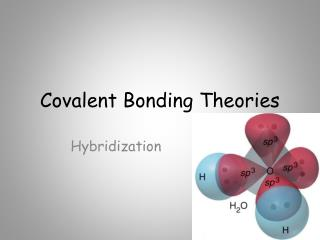DownloadDownload PresentationCovalent Bonding Theories

# Covalent Bonding Theories

Download Presentation## Covalent Bonding Theories

- - - - - - - - - - - - - - - - - - - - - - - - - - - E N D - - - - - - - - - - - - - - - - - - - - - - - - - - -
##### Presentation Transcript

1. Covalent Bonding Theories Hybridization

2. Theories of Covalent Bonding Valence Bond (VB) Theory and Orbital Hybridization The Mode of Orbital Overlap and the Types of Covalent Bonds Molecular Orbital (MO)Theory and Electron Delocalization

3. Valence Bond Theory • Linus Pauling and others applied the principles of quantum mechanics to molecules • They reasoned that bonds between atoms would occur when the orbitals on those atoms interacted to make a bond • The kind of interaction depends on whether the orbitals align along the axis between the nuclei, or outside the axis

4. Orbital overlap and spin pairing in three diatomic molecules. Hydrogen, H2 Hydrogen fluoride, HF Fluorine, F2

5. Valence Bond Theory – Hybridization • One of the issues that arises is that the number of partially filled or empty atomic orbitals did not predict the number of bonds or orientation of bonds • C = 2s22px12py12pz0 would predict two or three bonds that are 90° apart, rather than four bonds that are 109.5° apart

6. Bond Angles in Carbon Compounds electron configuration = 1s22s22p2 2p orbitals with one electron in each. Can p orbitals with one electron in each find the place where the 3rd p orbital should be? Orbitals with one electron in each will overlap to form single bonds. If they can, the bond angles should be 90o. But…the bond angles are 109.5o!

7. To adjust for these inconsistencies, it was postulated that the valence atomic orbitals could hybridizebefore bonding took place • one hybridization of C is to mix all the 2s and 2p orbitals to get four orbitals that point at the corners of a tetrahedron

8. Hybrid Orbitals • The number of standard atomic orbitals combined = the number of hybrid orbitals formed • combining a 2s with a 2p gives two 2sp hybrid orbitals • H cannot hybridize!! • its valence shell only has one orbital • The number and type of standard atomic orbitals combined determines the shape of the hybrid orbitals • The particular kind of hybridization that occurs is the one that yields the lowest overall energy for the molecule

9. Carbon Hybridizations Unhybridized    2p 2s sp hybridized     2sp 2p sp2 hybridized     2sp2 2p sp3 hybridized     2sp3

10. sp3 Hybridization • Atom with four electron groups around it • tetrahedral geometry • 109.5° angles between hybrid orbitals • Atom uses hybrid orbitals for all bonds and lone pairs

11. Bonding with Valence Bond Theory • According to valence bond theory, bonding takes place between atoms when their atomic or hybrid orbitals interact • “overlap” • To interact, the orbitals must either be aligned along the axis between the atoms, or • The orbitals must be parallel to each other and perpendicular to the interatomic axis

12. Methane Formation with sp3 C

13. Ammonia Formation with sp3 N

14. Orbital Diagrams of Bonding • “Overlap” between a hybrid orbital on one atom with a hybrid or nonhybridized orbital on another atom results in a s bond • “Overlap” between unhybridized p orbitals on bonded atoms results in a p bond

15. CH3NH2 Orbital Diagram s s s s s s s sp3 C sp3 N         s s s s s      1s H 1s H 1s H 1s H 1s H

16. Formaldehyde, CH2O Orbital Diagram p   p C p O s sp2 C sp2 O       s s   1s H 1s H

17. The sp2 hybrid orbitals in BF3.

18. Hybrid orbitals overlap to form a s bond. Unhybridized p orbitals overlap to form a p bond.

19. HCN Orbital Diagram 2p     p C p N s sp C sp N     s  1s H

20. sp3d • Atom with five electron groups around it • trigonal bipyramid electron geometry • Seesaw, T–Shape, Linear • 120° & 90° bond angles • Use empty d orbitals from valence shell • d orbitals can be used to make p bonds

21. Step 1 Step 2 Step 3 The conceptual steps from molecular formula to the hybrid orbitals used in bonding. Molecular shape and e- group arrangement Molecular formula Lewis structure Hybrid orbitals

22. Molecular Orbital Theory • In MO theory, we apply Schrödinger’s wave equation to the molecule to calculate a set of molecular orbitals • in practice, the equation solution is estimated • we start with good guesses from our experience as to what the orbital should look like • then test and tweak the estimate until the energy of the orbital is minimized • In this treatment, the electrons belong to the whole molecule – so the orbitals belong to the whole molecule • delocalization